# Attenuator Dissipation

On this page we will show you the equations for calculating the fractional dissipation of the individual resistors in an attenuator. As of October 2015, there is no other page on the world-wide web that provides this content, so it must be an esoteric topic, or something that designers ignore at their own peril!

Tee attenuator

Pi attenuator

Reflection attenuator

Bridged tee attenuator

The overall dissipation calculation for a lumped-element attenuator seems almost trivial, what ever you put in, minus what comes out is what is dissipated by the resistor network. However, the resistors in the pi or tee network do not share the burden of heat equally, you need to consider "R1A" could get hotter than "R1B" (see figure below), even though they have the same ohm value. The resistor that is closest to the power generator gets hit with the most power; if this is a surprise to you, perhaps you should change careers, maybe become a microwave vendor rep or become a "product assurance engineer" at a big and wasteful company. If you are designing an attenuator for high power, unless these resistors are located incredibly close to each other, you'll need to think about the dissipation in each resistor. It's a nasty algebra problem that we've solved for you!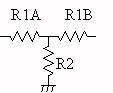# Tee attenuator

We solved the fractional dissipation in terms of R1 and Z0. Note that R1A and R1B are different resistors, but of identical value R1. Here's the equations: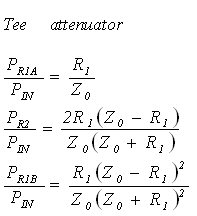Below are plots of the fractional dissipation of the three resistors in a tee attenuator, versus attenuation value, normalized to an input power of unity (one watt). In order to solve for actual dissipation as a function of input power, you need to multiply the curves by input power. Let's look at the curves... up to about 6 dB, the dissipation is approximately equal in R1A and R2. At infinite attenuation, the first resistor R1A gets all the dissipation. R1B never dissipates much of the power load at any attenuation value, which makes sense because it's at the attenuator's output.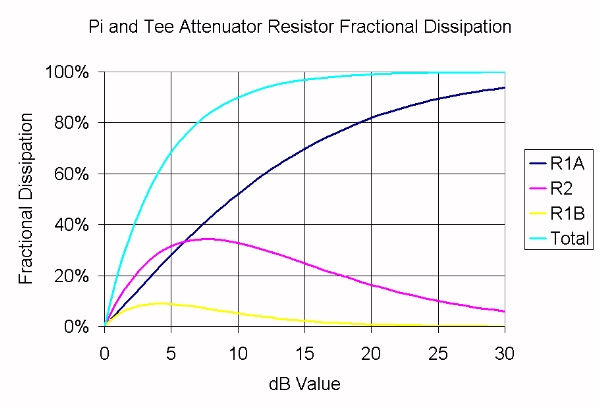# Pi attenuator

Funny thing about the pi attenuator... although the resistor values are very different than those of the tee attenuator, the fractional dissipation is exactly the same! The figure below explains why R1A dissipates the same in a tee and a pi, the remaining two resistors can be shown to have equivalent dissipation as well.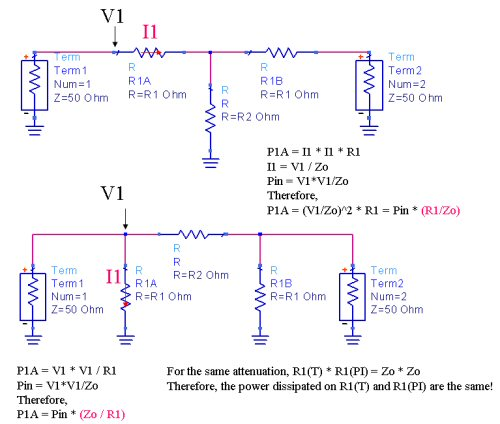So if you were lazy, you could stop here and never calculate the pi resistor fractional dissipation equations and just substitute the results of the tee-type attenuator versus attenuation. Not us! We've solved the equations for fractional dissipation for the pi attenuator, below, but we won't repeat the plot because it would be the same.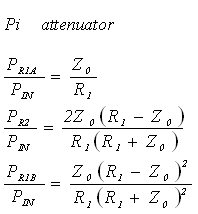It's time for another Microwaves101 rule of thumb! This applies to both tee and pi attenuator: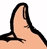For a three dB pad (50% power dissipation), the input resistor must be sized to handle 20% of the maximum intended input power (thanks for the correction, Dave!) For a ten dB pad (90% power dissipation), the input resistor must be sized to handle 1/2 of the maximum intended input power. For a 20 dB pad (99% power dissipation), size it for 80% of the maximum input power. For higher attenuation values, size the input resistor for the full RF input power.

# Reflection attenuator

Below is a plot of the fractional dissipation of resistors in a reflection attenuator. The math is trivial, theoretically the two resistors split the dissipated power 50/50.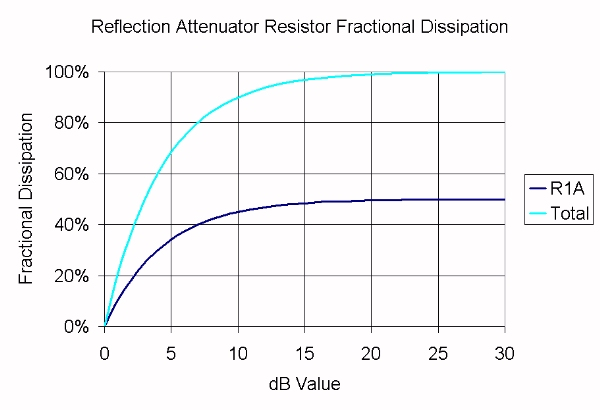# Bridged tee attenuator

New for October 2015, by request! The bridged tee attenuator is unusual, in that the component values and their RF currents are not so easy to calculate. Let's start by naming the individual resistors. Note that the schematic below was done in Microwave Office,  the circuit is set up for harmonic balance analysis with 1 watt input on the left (30 dBm) and the right side is terminated in 50 ohms.  In the figure, Z1A and Z1B are two resistors with value Z0, or 50 ohms in a 50 ohm system. Hey, we should have called them Z0A and Z0B, doh!  The equations calculate R1 and R2 from the value of "DB".

###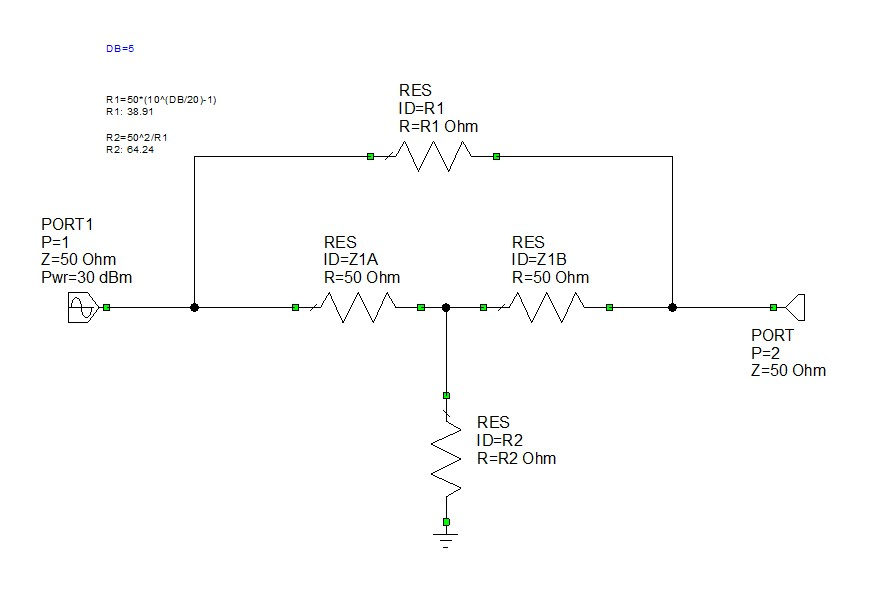The next step might be applying Kirchhoff's Law: the sum of all currents into a node is zero. Note that Mr. Kirchhoff is in ourMicrowaves Hall of Fame! From this you can derive a set of equations and try to solve for the current through each resistor as a function of Vin/Vout. We tried that, it was quite a mess and beyond the limited skill set of a United Airlines passenger sitting between two fat people.  Then we noticed two huge shortcuts to the analysis, when we examined the dissipations using harmonic balance: there is no dissipation in Z1B, and R1 and R2 have identical dissipations (246.1 mW in the plot below). The plot is for a 5 dB attenuator. Thanks, Microwave Office! Here's a Microwaves101 page on harmonic balance analysis that  could use some content...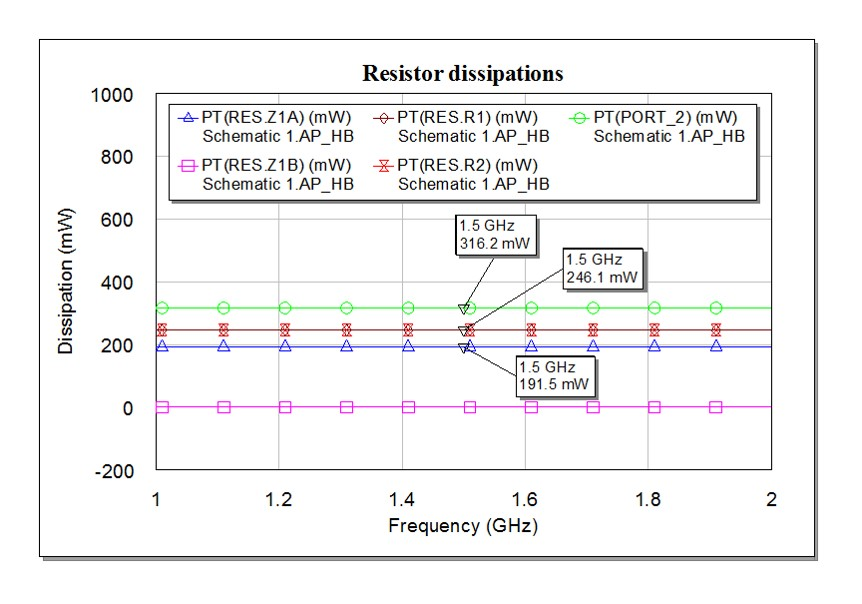How can Z1B have zero dissipation? Simply put, the voltage dividers to the left and right of it always provide the same voltage value: R2/(Z0+R2)=Z0/(R1+Z0). Maybe can prove that to yourself for homework, noting that R2=Z0^2/R1. We tried and failed to prove it, but we can believe it because harmonic balance doesn't lie.  Engineering is all about the solution, not how it to prove or derive it.

If Z1B has no dissipation, there is no RF current through it. Furthermore, you can removed it from the schematic and the bridged tee becomes an L-pad attenuator. Why is it even there? Without Z1B the attenuator is not matched to Z0 looking in the reverse direction.

Once these tricks have been revealed it is a simple matter to calculate the fractional dissipations.  Note that at infinite attenuation, all dissipation occurs in Z1A (R2 is zero ohms in this case and dissipates nothing, R1 is infinite and also dissipates nothing). Thus you will need to size Z1A and Z1B to handle the entire load. R1 and R2 never see more than 25% of the input power. Remember, Z1B will dissipate power if you turn the attenuator around.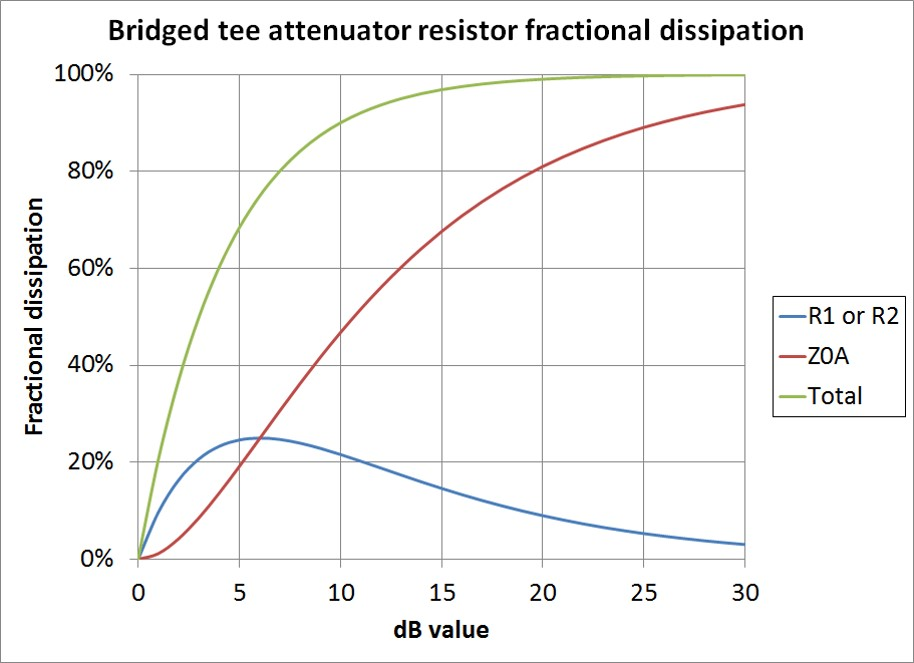Update November 2015: We now have the the intermediate steps to deriving these equations using Kirchhoff's Law.